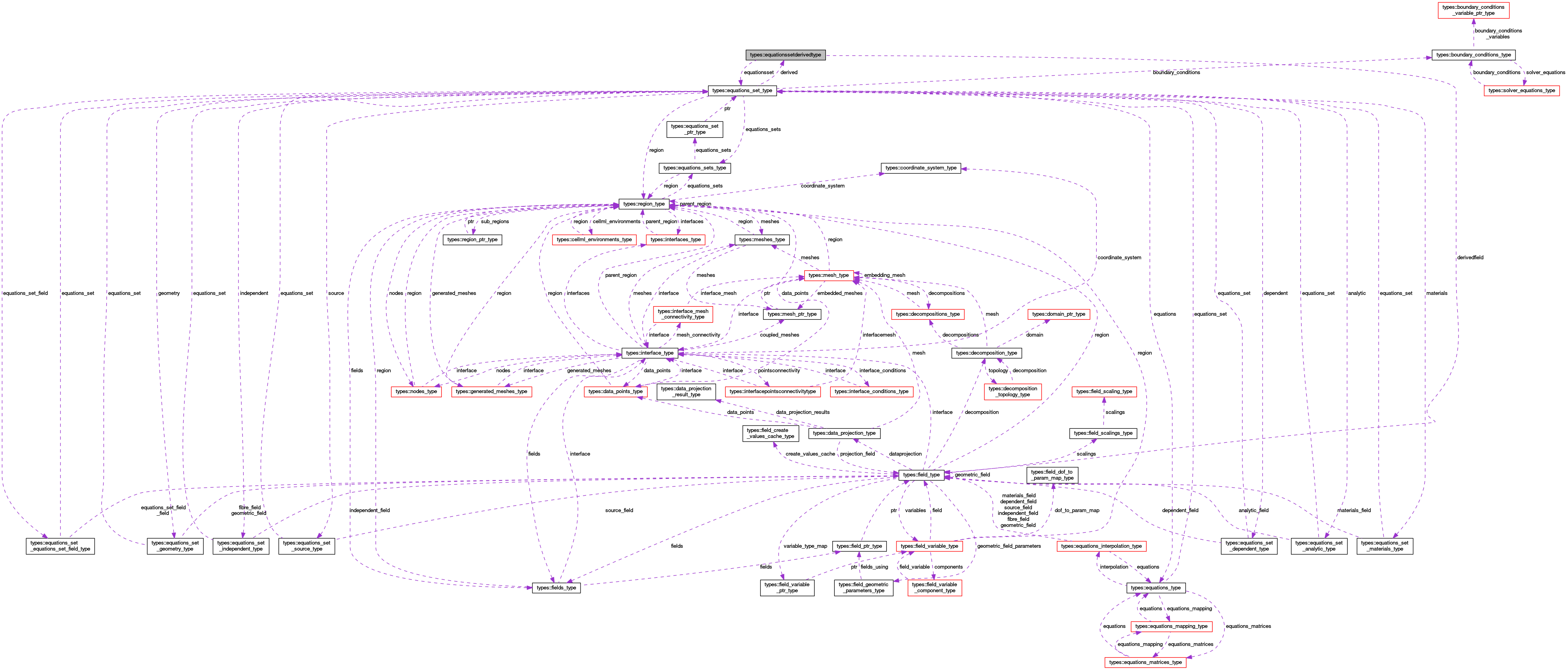OpenCMISS-Iron Internal API Documentation
types::equationssetderivedtype Type Reference

Contains information on the derived variables for the equations set, eg. stress or strain. More...

Collaboration diagram for types::equationssetderivedtype:[legend]

## Public Attributes

type(equations_set_type), pointer equationsset
A pointer back to the equations set. More...

logical derivedfinished
Is .TRUE. if the derived variables for the equations set have finished being created, .FALSE. if not. More...

logical derivedfieldautocreated
Is .TRUE. if the derived field has been or will be auto created by the equations set setup, .FALSE. if it was already created. More...

type(field_type), pointer derivedfield
A pointer to the derived field for the equations set. More...

integer(intg) numberofvariables
The number of derived variables used. More...

integer(intg), dimension(:), allocatable variabletypes
variableTypes(DERIVED_TYPE) is zero if the derived type is not used, otherwise it is the field variable type in the derived field for the derived variable type More...

## Detailed Description

Contains information on the derived variables for the equations set, eg. stress or strain.

Definition at line 1897 of file types.f90.

## Member Data Documentation

 type(field_type), pointer types::equationssetderivedtype::derivedfield

A pointer to the derived field for the equations set.

Definition at line 1901 of file types.f90.

 logical types::equationssetderivedtype::derivedfieldautocreated

Is .TRUE. if the derived field has been or will be auto created by the equations set setup, .FALSE. if it was already created.

Definition at line 1900 of file types.f90.

 logical types::equationssetderivedtype::derivedfinished

Is .TRUE. if the derived variables for the equations set have finished being created, .FALSE. if not.

Definition at line 1899 of file types.f90.

 type(equations_set_type), pointer types::equationssetderivedtype::equationsset

A pointer back to the equations set.

Definition at line 1898 of file types.f90.

 integer(intg) types::equationssetderivedtype::numberofvariables

The number of derived variables used.

Definition at line 1902 of file types.f90.

 integer(intg), dimension(:), allocatable types::equationssetderivedtype::variabletypes

variableTypes(DERIVED_TYPE) is zero if the derived type is not used, otherwise it is the field variable type in the derived field for the derived variable type

Definition at line 1903 of file types.f90.